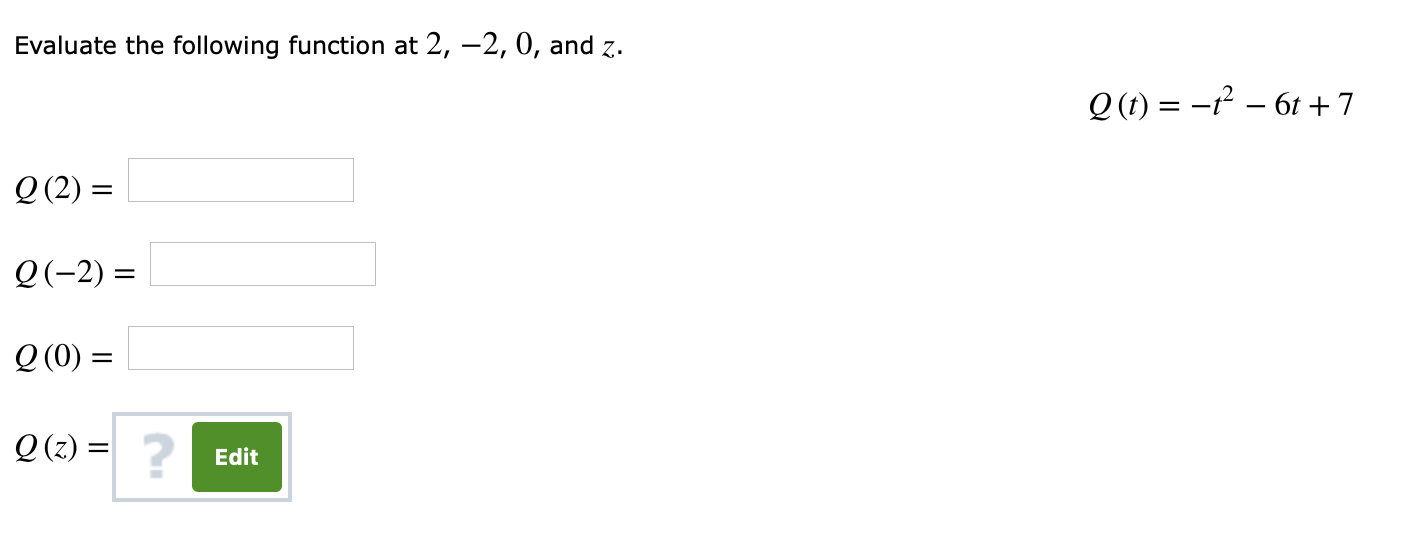# Evaluate the following function at 2, -2, 0, and z.2(2)Q(-2)20)22)Q (z)2Edit

Question
1 viewshelp_outlineImage TranscriptioncloseEvaluate the following function at 2, -2, 0, and z. 2(2) Q(-2) 20) 22) Q (z) 2 Edit fullscreen
check_circle

Step 1

Substitute t = 2 in Q (t) = –t^2–6t+7 and evaluate the value of the function as follows.

Step 2

Substitute t = –2 in Q (t) = –t^2–6t+7 and evaluate the value of the function as follows.

Step 3

Substitute t = 0 in Q (t) = –t^2–6t+7 and evaluate the...

### Want to see the full answer?

See Solution

#### Want to see this answer and more?

Solutions are written by subject experts who are available 24/7. Questions are typically answered within 1 hour.*

See Solution
*Response times may vary by subject and question.
Tagged in

### Other